# Problem 52819. Easy Sequences 30: Nearly Pythagorean Triangles

A Nearly Pythagorean Triangle (abbreviated as "NPT'), is an integer-sided triangle whose square of the longest side, which we will call as its 'hypotenuse', is 1 more than the sum of square of the shorter sides. This means that if c is the hypotenuse and a and b are the shorter sides,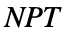, satisfies the following equation: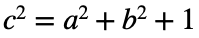where: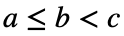The smallestis the triangle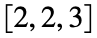, with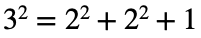. Other examples are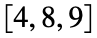,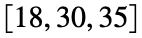, and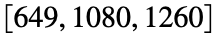.
Unfortunately, unlike Pythagorean Triangles, a 'closed formula' for generating all possible's, has not yet been discovered, at the time of this writing. For this exercise, we will be dealing with's with a known ratio of the shorter sides: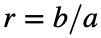.
Given the value of r, find thewith the second smallest perimeter. For example for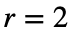, that is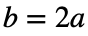, the smallest perimeter is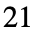, while the second smallest perimeter is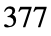, for thewith dimensions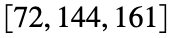. Please present your output as vector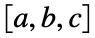, where a is the smallest side of the, and c is the hypotenuse.

### Solution Stats

42.65% Correct | 57.35% Incorrect
Last Solution submitted on Oct 28, 2023

### Community Treasure Hunt

Find the treasures in MATLAB Central and discover how the community can help you!

Start Hunting!